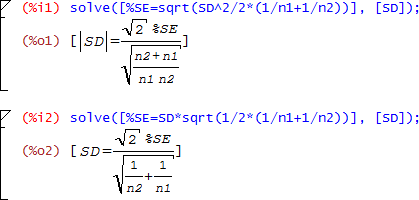## SE of ∆ [General Sta­tis­tics]

Dear Detlew,

» I think all the confusion comes from that sigmaw, sigmad, sigmadelta values including their estimates which are used by all the authors cited within this thread in a different meaning.

Quite possible.

» I'm not able to figure out who is who, what is what. Sorry.

F**ing terminology.

» The only thing I'm convinced of is that your formula (2) above is correct.
» If you write the confidence interval for the BE decision as
» PE(T-R) +- SD(d)*tval(0.95, df)

Exactly.

» The rest of your algebra is straight forward.
» And correct if you ask me.

THX. Now three people agree. I even didn’t trust my rusty algebra and asked Maxima for help:» BTW: the formula (2) is not the error term in the 2×2×2 crossover.

How would you call it? We use $$(3)$$ in PowerTOST’s BE_CI.R line 30:

sqrt(mse*ades$bkni*nc) where for design = "2x2" ades$bkni is 0.5 and nc is sum(1/n+1/n).
If we agree that $$\small{\widehat{\sigma}_\textrm{w}=\sqrt{MSE}}$$ * we end up with $$(2)$$:

      For the special case of a two-treatment, two-period crossover study in which $$\small{n_\textrm{1}}$$ subjects receive the test formulation in period one and the reference formulation in period two, while $$\small{n_\textrm{2}}$$ subjects receive the reference formulation in period one and the reference formulation in period two, the unbiased estimator is given by $$\small{Est.=\frac{\left(\bar{X}_\textrm{T1}+\bar{X}_\textrm{T2} \right)}{2}-\frac{\left(\bar{X}_\textrm{R1}+\bar{X}_\textrm{R2} \right)}{2}}$$ where
      $$\small{\bar{X}_\textrm{T1}=}$$ the observed mean of the $$\small{n_\textrm{1}}$$ observations of the test formulation in period one.
      $$\small{\bar{X}_\textrm{T2}=}$$ the observed mean of the $$\small{n_\textrm{2}}$$ observations of the test formulation in period two.
      $$\small{\bar{X}_\textrm{R1}=}$$ the observed mean of the $$\small{n_\textrm{2}}$$ observations of the reference formulation in period one.
      $$\small{\bar{X}_\textrm{R2}=}$$ the observed mean of the $$\small{n_\textrm{1}}$$ observations of the reference formulation in period two.
The standard error of this estimator is $$\small{SE=s\sqrt{\frac{1}{2}\left (\frac{1}{n_\textrm{1}}+\frac{1}{n_\textrm{2}} \right)}}$$ where […] $$\small{s}$$ is the square root of the “error” mean square from the crossover analysis of variance, based on $$\small{\nu}$$ degrees of freedom.

• Schuirmann DJ. A comparison of the Two One-Sided Tests Procedure and the Power Approach for Assessing the Equivalence of Average Bioavailability. J Pharmacokin Biopharm. 1987; 15(6): 657–80. doi:10.1007/BF01068419.

Dif-tor heh smusma 🖖Helmut SchützThe quality of responses received is directly proportional to the quality of the question asked. 🚮
Science QuotesIng. Helmut Schütz